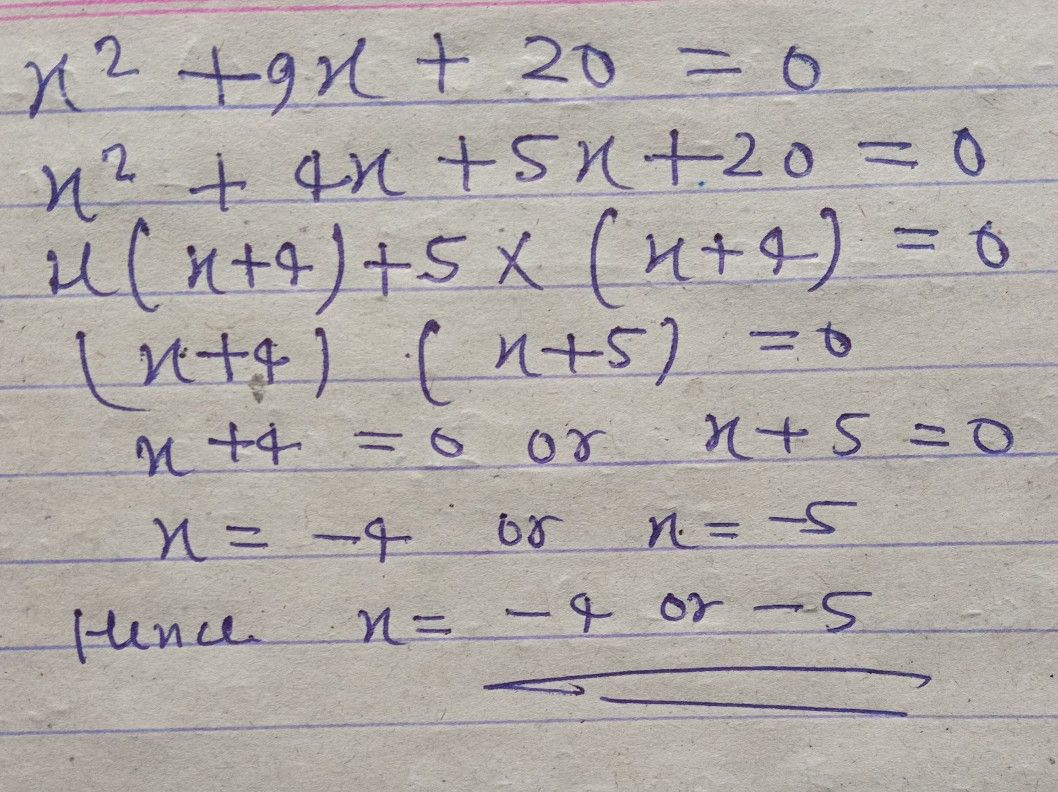Symbol
Problemthe mathematical sentences below to answer the questions that $01lo8y$ $2x^{2}+7s+5>0$ $15-6h^{2}=10$ $r^{2}+10r\leq -16$ $4x^{2}-25=0$ $3w^{2}+12w\geq$ $m^{2}=6m-7$ $x^{2}+9x+20$ $=0$ $2t^{2}<21-9t$ $tions$ $1$ Which of the given mathematical sentences are quadratic equations? Ans. $2$ How do you describe quadratic equations? Ans. $3$ Which of the given mathematical sentences are not quadratic equations? Why? Ans. $4$ How would you describe those mathematical sentences which are not quadratic equ $4$ Ans. How are they different from those equations which are quadratic? $A$ Ans.
7th-9th grade
Other
Search count: 122
SolutionQanda teacher - varsha
this is how to do it